# DS博客作业03--树

| 这个作业属于哪个班级 | 数据结构--网络2011/2012 |
| ---- | ---- | ---- |
| 这个作业的地址 | DS博客作业03--树 |
| 这个作业的目标 | 学习树结构设计及运算操作 |
| 姓名 | 韩龙飞 |

# 0.PTA得分截图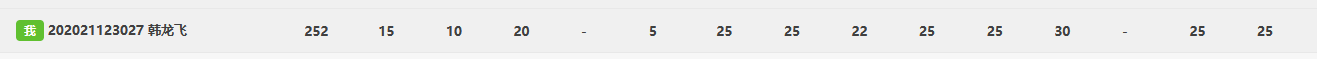# 1.本周学习总结（5分）

## 1.1 二叉树结构

### 1.1.1 二叉树的2种存储结构

#### Ⅰ.顺序存储结构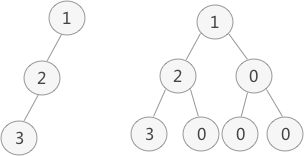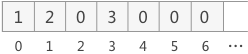typedef ElemType SqBinTree[MaxSize]


• 插入删除操作不方便
• 对于完全二叉树顺序存储结构很适合
• 如果需要的空结点太多，会导致空间开辟过多

#### Ⅱ.链式存储结构

lchild和rchild分别表示左指针域和右指针域，分别用于存储左右孩子结点的存储地址。这种链式存储结构通常简称为二叉链。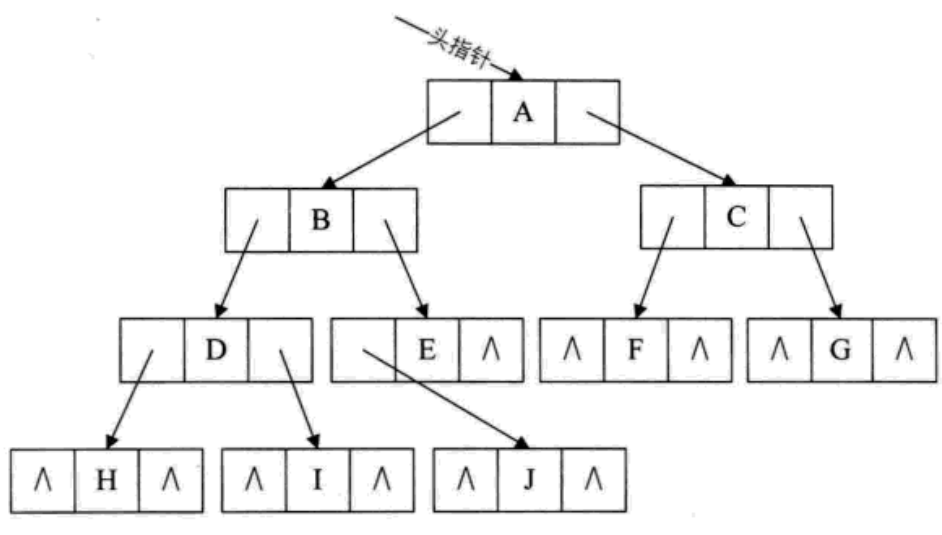typedef struct node
{
ElemType data;
struct node* lchild;
struct node* rchild;
}BTNode;


• 相较于顺序存储结构节省空间
• 再二叉链中访问一个结点的孩子方便

### 1.1.2 二叉树的构造

#### Ⅰ.利用先序和中序序列构造二叉树

BinTree CreatBTree(char a[], char b[], int n)
{
BinTree T;
int i;
if (!n)
return NULL;
else
{
T = new TNode;
T->Data = a;
for (i = 0; i < n; i++)
{
if (a == b[i])
break;
}
T->Left = CreatBTree(a + 1, b, i);
T->Right = CreatBTree(a + i + 1, b + i + 1, n - i - 1);
return T;
}
}


### 1.1.3 二叉树的遍历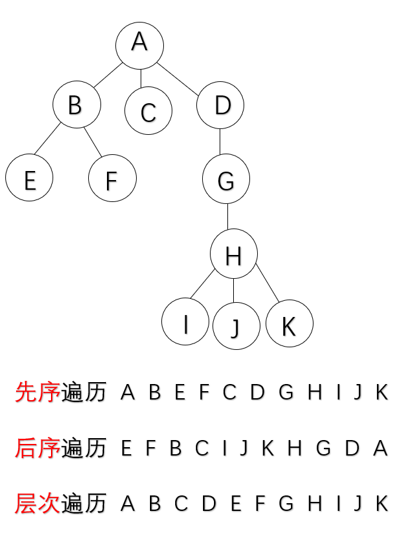#### Ⅰ.先序遍历

void PreOrder(BTNode* b)
{
if (b != NULL)
{
cout << b->data;
PreOrder(b->lchild);
PreOrder(b->rchild);
}
}


#### Ⅱ.中序遍历

void InOrder(BTNode* b)
{
if (b != NULL)
{
InOrder(b->lchild);
cout << b->data;
InOrder(b->rchild);
}
}



#### Ⅲ.后序遍历

void PostOrder(BTNode* b)
{
if (b != NULL)
{
PostOrder(b->lchild);
PostOrder(b->rchild);
cout << b->data;

}
}



### 1.1.4 线索二叉树

#### Ⅰ.线索二叉树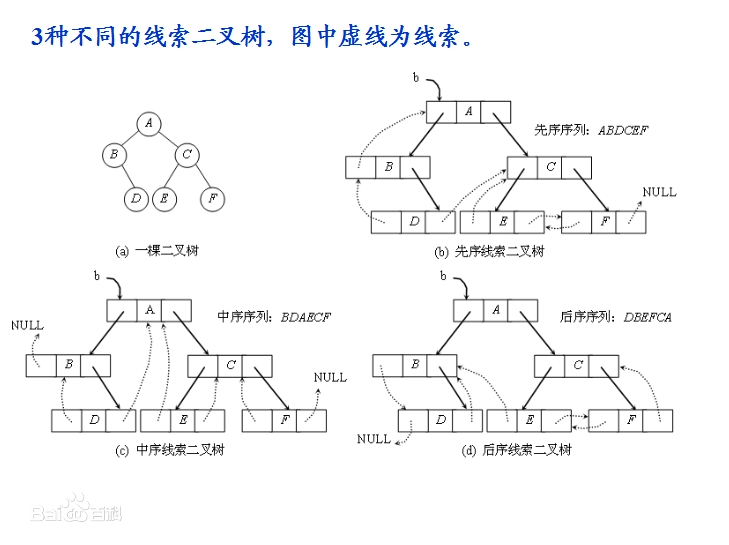typedef struct node
{
ElemType data；
int ltag,rtag;//增加的线索标记
struct node* lchild;
struct node* rchild;
}


binThiTree* preTreeNode(binThiTree* q) {
binThiTree* cur;
cur = q;
if (cur->LTag == true) {
cur = cur->lchild;
return cur;
}
else{
cur = cur->lchild;//进入左子树
while (cur->RTag == false) {
cur = cur->rchild;
}//找到左子树的最右边结点
return cur;
}
}


binThiTree* rearTreeNode(binThiTree* q) {
binThiTree* cur = q;
if (cur->RTag == true) {
cur = cur->rchild;
return cur;
}
else {
//进入到*cur的右子树
cur = cur->rchild;
while (cur->LTag == false) {
cur = cur->lchild;
}
return cur;
}
}


### 1.1.5 二叉树的应用--表达式树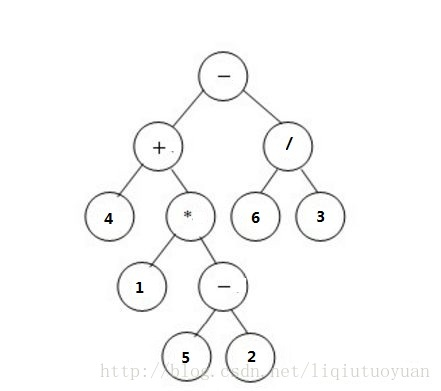PTree CreatExpTree()
{
char data;
PTree T;
Pstack P = CreatStack();

cout << "请输入后缀表达式：";
while (cin >> data)
{
if ('a'<= data && 'z' >= data)
{
T = (PTree)malloc(sizeof(Tree));
T->data = data;
T->left = NULL;
T->right = NULL;
Push(P, T);
}
else
{
T = (PTree)malloc(sizeof(Tree));
T->data = data;
T->right = Pop(P);
T->left = Pop(P);
Push(P, T);
}
}
return Pop(P);    //返回树的根节点
}


## 1.2 多叉树结构

### 1.2.1 多叉树结构

#### Ⅰ.孩子兄弟链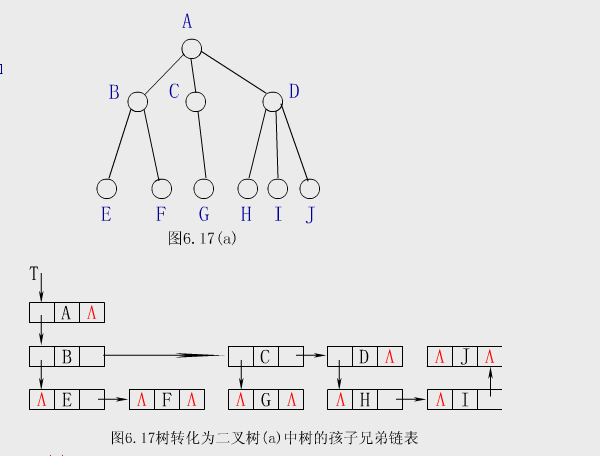typedef struct tnode
{
ElemType data;
struct tnode* hp;//指向兄弟
struct tnode* vp;//指向孩子
};


#### Ⅱ.孩子链

typedef struct node
{
ElemType data;
struct node* sons[MaxSons];
};


### 1.2.2 多叉树遍历

Ⅰ.先序遍历递归算法

void PreOrder(BTNode* b)
{
if (b != NULL)
{
cout << b->data;
PreOrder(b->lchild);
PreOrder(b->rchild);
}
}


Ⅱ.先序遍历非递归算法

void preorder(BTNode* b)
{
BTNode* p;
SQStack* st;
InitStack(st);
if (b != NULL)
{
Push(st, b);
while (!StackEmpty(st))
{
Pop(st, p);
cout << p->data << endl;
if (p->rchild != NULL)
Push(st.p->rchild);
if (p->lchild != NULL)
Push(st.p->lchild);
}
}
DestroyStack(st);
}


## 1.3 哈夫曼树

### 1.3.1 哈夫曼树定义

WPL=∑WiLi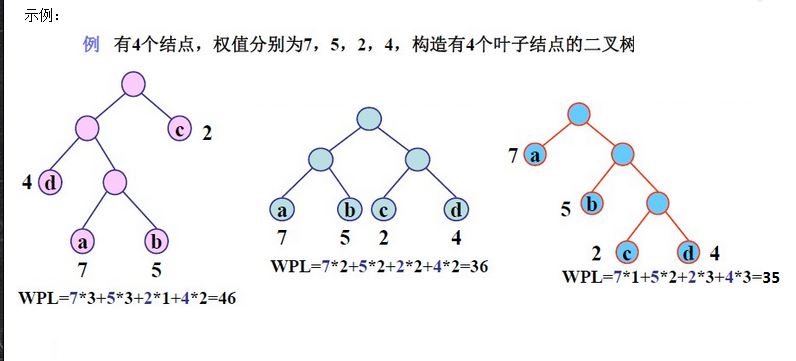### 1.3.2 哈夫曼树的结构体

/*顺序结构*/
typedef struct node{
char data;//节点值
double weight;//权重
int parent;//双亲节点
int lchild;//左孩子节点
int rchild;//右孩子节点
}HTNode;
/*链式结构*/
typedef struct node
{
char data;            //结点数据
double weight;         //权重
struct node* parent; //双亲节点
struct node* lchild; //左孩子
struct node* rchild; //右孩子
struct node* next;    //指向下一个节点
}HTNode;


### 1.3.3 哈夫曼树构建及哈夫曼编码

(1) 将w1、w2、…，wn看成是有n 棵树的森林(每棵树仅有一个结点)；
(2) 在森林中选出两个根结点的权值最小的树合并，作为一棵新树的左、右子树，且新树的根结点权值为其左、右子树根结点权值之和；
(3)从森林中删除选取的两棵树，并将新树加入森林；
(4)重复(2)、(3)步，直到森林中只剩一棵树为止，该树即为所求得的哈夫曼树。
※为了使得到的哈夫曼树的结构尽量唯一，通常规定生成的哈夫曼树中每个结点的左子树根结点的权小于等于右子树根结点的权

![](https://img2020.cnblogs.com/blog/2160478/202105/2160478-20210508145604551-1792288385.png)

void CreateHT(HTNode ht[], int n0)
{
int i, k, lnode, rnode;
double min1, min2;
for (i = 0; i < 2 * n0 - 1; i++)
ht[i].parent = ht[i].lchild = ht[i].rchild = -1;//所有结点域置初值
for (i = n0; i <= 2 * n0 - 2; i++)
{
min1 = min2 = 32767;
lnode = rnode = -1;
for (k = 0; k <= i - 1; k++)//在ht[0……i-1]中找权最小的两个结点
{
if (ht[k].weight < min1)//只在尚未构造二叉树的结点中查找
{
min2 = min1;
rnode = lnode;
min1 = ht[k].weight;
lnode = k;
}
else if (ht[k].weight < min2)
{
min2 = ht[k].weight;
rnode = k;
}
}
ht[i].weight = ht[lnode].weight + ht[rnode].weight;
ht[i].lchild = lnode;
ht[i].rchild = rnode;
ht[lnode].parent = i;
ht[rnode].parent = i;
}
}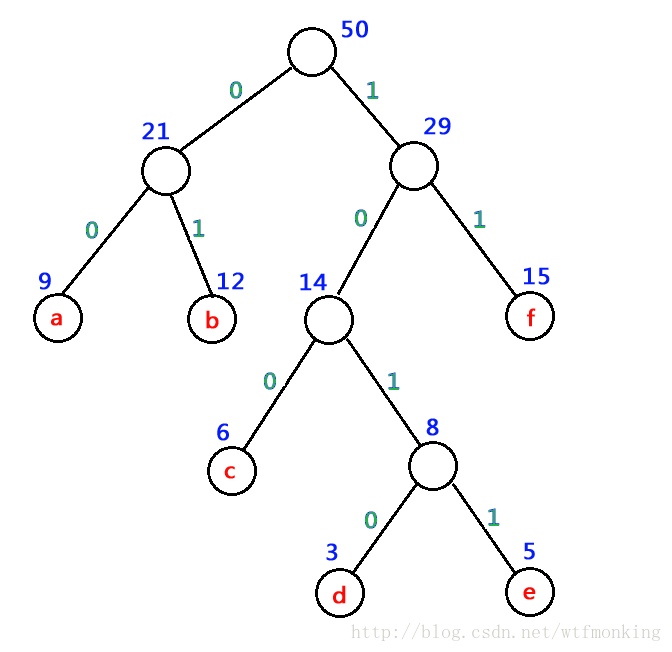a 的编码为：00
b 的编码为：01
c 的编码为：100
d 的编码为：1010
e 的编码为：1011
f 的编码为：11

## 1.4 并查集

/*结构体*/
typedef struct node
{
int data;//结点对应的编号
int rank;//结点秩，子树的高度
int parent;//结点对应的双亲下表
}UFSTree;

/*初始化*/
void MakeSet(UFSTree t[], int n)
{
for (int i = 1; i <= n; i++) {
t[i].data = i;
t[i].rank = 0;
t[i].parent = i;
}
}

/*查找*/
int FindSet(UFSTree t[], int x)//找双亲
{
if (x != t[x].parent)return FindSet(t, t[x].parent);
else return x;
}

/*合并*/
void Union(UFSTree t[],int x, int y)
{
x = FindSet(t, x);
y = FindSet(t, y);
if (t[x].rank > t[y].rank)t[y].parent = x;
else {
t[x].parent = y;
if (t[x].rank == t[y].rank)
t[y].rank++;
}
}


## 1.5.谈谈你对树的认识及学习体会。

• 与先前学习的线性结构不同，树是一个非线性结构
• 树这一章的递归非常的多，虽然递归的调试难度比较大，但代码量却很少，而且顺着思路一步步的思考下去也是一件很有意思的事情
• 我认为树对于局部变量和全局变量的区分度更高，不能再像原来一样混淆不清的使用了，就像引用型，稍不注意就会混淆使用，导致输出结果的错误。

# 2.PTA实验作业（4分）

## 2.1 二叉树

### 2.1.1 解题思路及伪代码

BinTree CreateBtree(string str, int i)
BinTree btNode = new Bnode;
btNode->data = str[i];
int t = 2 * i, count = 0;
while t > 1
t /= 2
count++
end while
btNode->level=count-1
if 2*i大于字符串的长度或者str[2*i]为井号
左子树为空
else
左子树往下递归
end if
if 2*i+1大于字符串的长度或者str[2 * i+1]为井号
右子树为空
else
右子树往下递归


int CntWpl(BinTree bt)
{
int result = 0;
if 左子树和右子树不为空
result += (bt->data - '0') * bt->level;
end if
if 左子树不为空
result += CntWpl(bt->left);
end if
if 右子树不为空
result += CntWpl(bt->right);
end if
return result
}


• 顺序存储结构建树
• 递归

## 2.2 目录树

### 2.2.1 解题思路及伪代码

/*这里只作插入的伪代码*/
void InsertTreeNode(DirTree& tr, DirTree node)

if tr没有孩子
直接将node做第一个孩子，指针指向node;
返回;
end if
if tr第一个孩子的数据元素等于node的数据元素并且tr的第一个孩子的类型等于node的数据元素类型
tr=tr->firstchild;
return;
end if
if tr孩子类型为文件node类型为目录或者两个类型相同但node数据元素小于tr孩子数据元素
node的兄弟=tr的孩子
tr的孩子=node
指针指向node
return
end if
if 头没有兄弟
头兄弟等于node
指针指向node
end if
while 有兄弟并且兄弟的数据元素小于node数据元素并且类型都为目录
找到插入位置后插入数据
end while


• string库函数的使用
• 插入结点
• bool的使用

# 3.阅读代码（0--1分）

## 3.1 题目及解题代码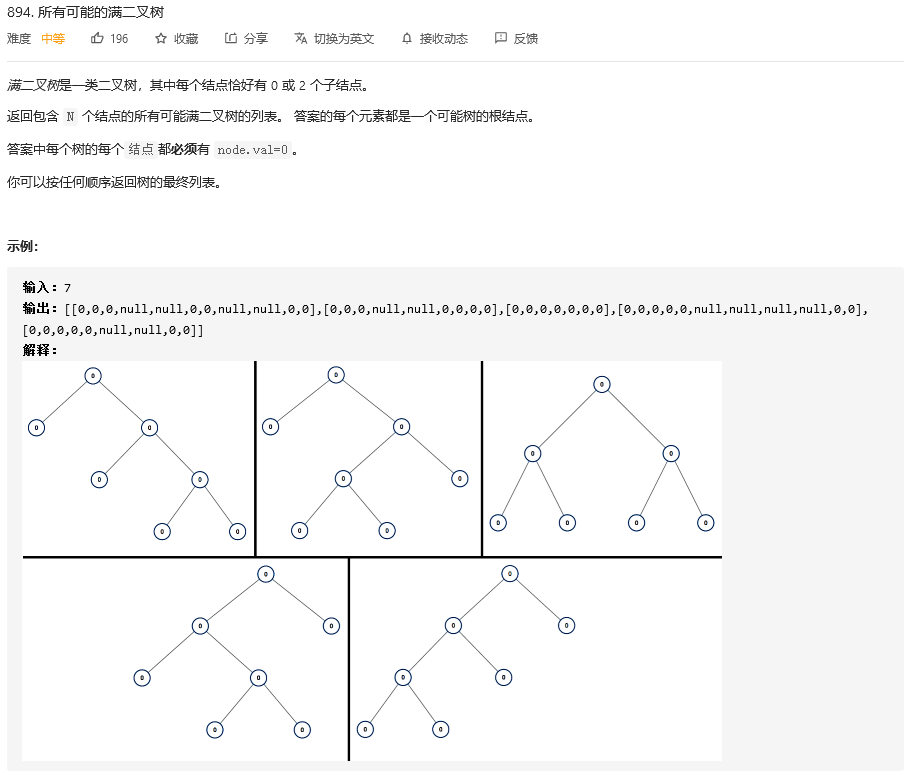Definition for a binary tree node.
struct TreeNode {
int val;
TreeNode *left;
TreeNode *right;
TreeNode(int x) : val(x), left(NULL), right(NULL) {}
};

class Solution {
vector<TreeNode*> temp;
public:
vector<TreeNode*> allPossibleFBT(int N) {
vector<TreeNode*> dp;
if(N & 1 == 0) return dp;
if(N == 1) {dp.push_back(new TreeNode(0));return dp;}
for(int i=1;i<=N-2;i+=2){
vector<TreeNode*> left = allPossibleFBT(i);
vector<TreeNode*> right = allPossibleFBT(N-1-i);
for(int j=0;j<left.size();++j){
for(int k=0;k<right.size();++k){
TreeNode *root = new TreeNode(0);
root->left = left[j];
root->right = right[k];
dp.push_back(root);
}
}
}
return dp;
}
};


## 3.3 分析该题目解题优势及难点。

posted @ 2021-05-02 12:40  箱推人  阅读(46)  评论(0编辑  收藏  举报